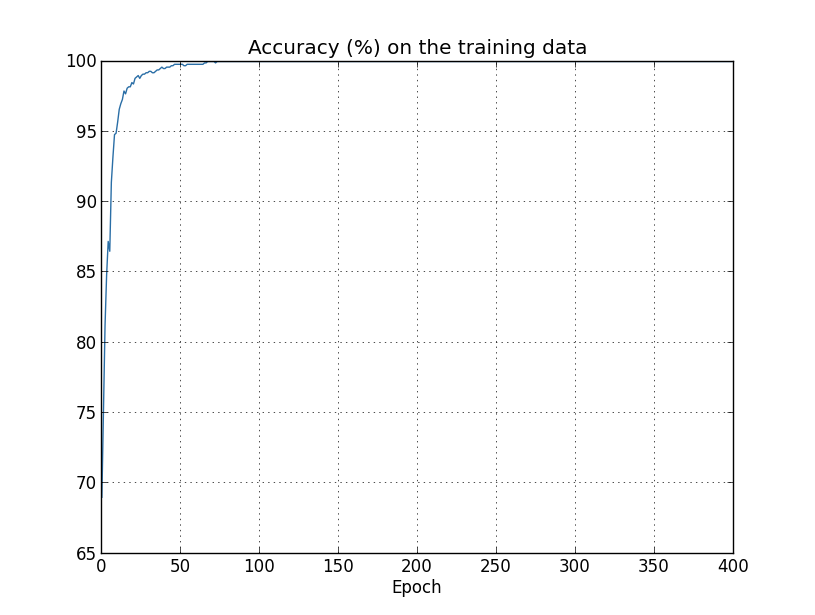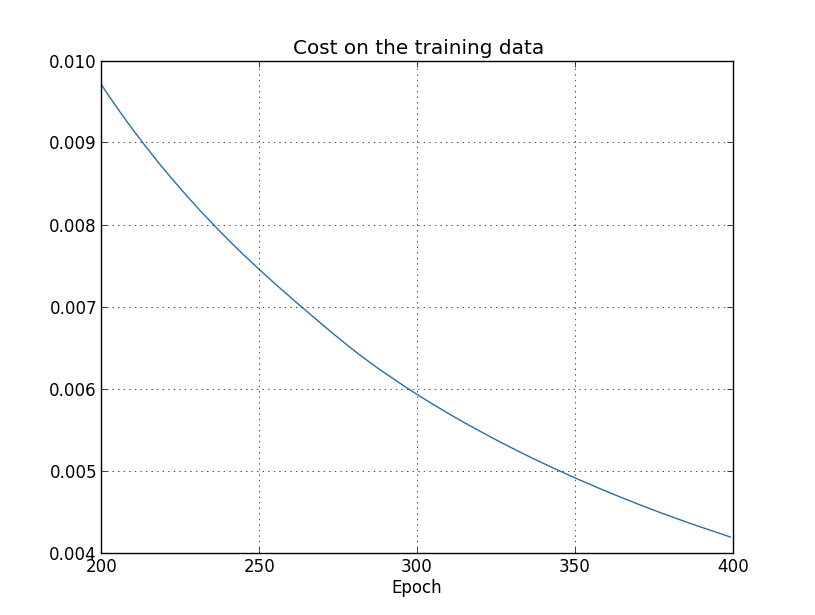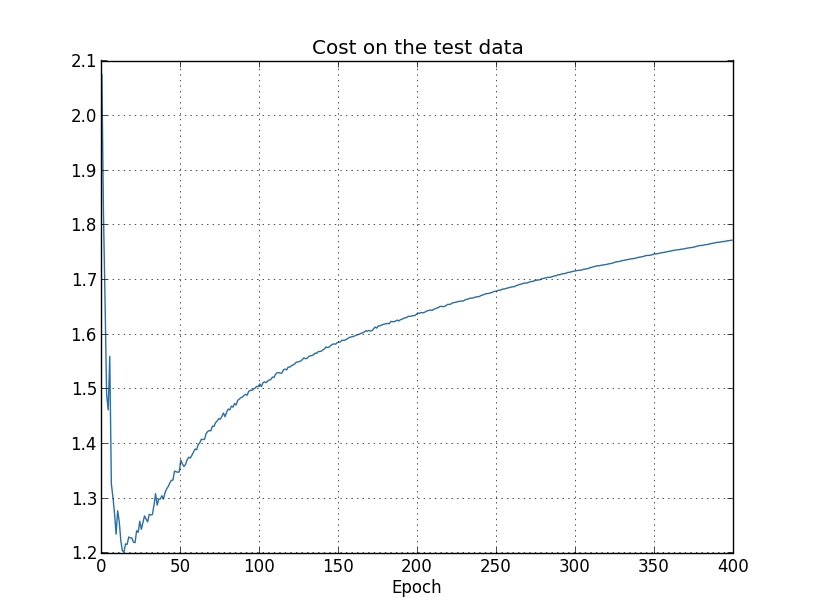# Neural Networks and Deep Learning（三·二）过拟合与正则化$\begin{eqnarray} C = C_0(w,x,y) + \lambda\Omega(w)\tag{1}\end{eqnarray}$

$\begin{eqnarray} C = C_0 + \frac{\lambda}{2n}\sum_w w^2,\tag{2}\end{eqnarray}$

(2)式的直观含义是，$\min C$的过程中，我不但希望损失函数本身$C_0$足够小，还希望网络的权重$w$也比较小，最好不要出现很大的$w$。如果$\lambda$越大，表示正则化越厉害，对大的$w$惩罚越严重。

$\begin{eqnarray}\frac{\partial C}{\partial w} & = & \frac{\partial C_0}{\partial w} + \frac{\lambda}{n} w \tag{3}\\ \frac{\partial C}{\partial b} & = & \frac{\partial C_0}{\partial b}.\tag{4}\end{eqnarray}$

$\begin{eqnarray}b & \rightarrow & b -\eta \frac{\partial C_0}{\partial b}.\tag{5}\end{eqnarray}$

$\begin{eqnarray} w & \rightarrow & w-\eta \frac{\partial C_0}{\partial w}-\frac{\eta \lambda}{n} w \tag{6}\\ & = & \left(1-\frac{\eta \lambda}{n}\right) w -\eta \frac{\partial C_0}{\partial w}. \tag{7}\end{eqnarray}$

$\begin{eqnarray} w \rightarrow \left(1-\frac{\eta \lambda}{n}\right) w -\frac{\eta}{m}\sum_x \frac{\partial C_x}{\partial w}, \tag{8}\end{eqnarray}$

$\begin{eqnarray}b \rightarrow b - \frac{\eta}{m} \sum_x \frac{\partial C_x}{\partial b},\tag{9}\end{eqnarray}$L1正则和L2正则类似，只不过换成了对$w$的一范数，损失函数如下：

$\begin{eqnarray} C = C_0 + \frac{\lambda}{n} \sum_w |w|.\tag{10}\end{eqnarray}$

$w$的权重更新公式如下，和(7)很类似，不细说了。

$\begin{eqnarray} w \rightarrow w' =w-\frac{\eta \lambda}{n} \mbox{sgn}(w) - \eta \frac{\partial C_0}{\partial w},\tag{11}\end{eqnarray}$

L1正则和L2正则都有一个权重衰减因子，虽然因子不同，但目的都是为了让$w$衰减，尽量小。他们的差别是L2衰减的是$w$的一个比例（公式(7)，$w\left(1 - \frac{\eta \lambda}{n} \right)$），而L1衰减的是一个固定值（公式(11)，$w-\frac{\eta \lambda}{n} \mbox{sgn}(w)$）。这就导致如果$w$本身很大的话，L1减的相对更少，而L2减的相对更多；如果$w$本身很小的的话，L1减的相对就更多，而L2减的相对更少。所以L1倾向于把小的$w$直接减到0，而对大的$w$不怎么减，而L2对所有$w$都一视同仁的减一定的比例。所以L1倾向于保留大的$w$，而直接把小的$w$正则为0，所以L1得到的非0权重可能更少，能得到稀疏模型，有利于特征选择。

Dropout

Dropout的做法和L1正则、L2正则很不一样，它不对损失函数进行修改，而是对网络结构进行修改。Dropout会在每个mini-batch训练时，随机删掉网络中一半的神经元（并不是真正的删除，而是暂时把对应的$w$设置为0）。它的效果相当于每次训练只用了一半的神经元，那么这次mini-batch和下次mini-batch相当于训练了不同的网络，最后预测时恢复所有的神经元。这样得到的预测结果相当于多个神经网络进行了average或者voting，使得预测结果更加鲁棒。比如有5个模型，其中3个模型预测对了，2个模型预测错了，做一下voting的话，就能得到正确的结果，而如果只是一个模型的话，如果刚好预测错了，那就错了。Dropout的另一个解释时，因为网络在训练时会随机删掉一半的节点，那么节点间的依赖关系就减弱了，迫使神经元依赖较少的信息也要得到比较好的预测结果，所以网络会更加鲁棒。Dropout在深层神经网络中，特别有用，能有效防止过拟合。不过也正因为此，dropout会增加训练收敛的时间，这是可以理解的。

This site uses Akismet to reduce spam. Learn how your comment data is processed.